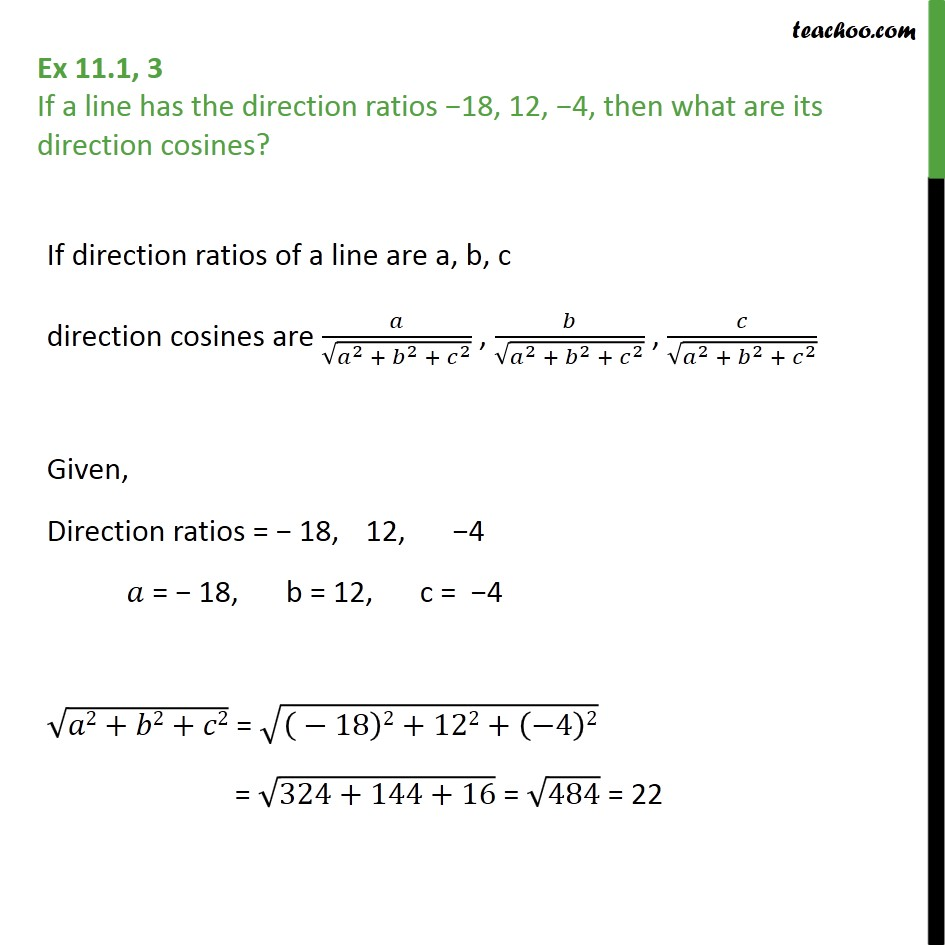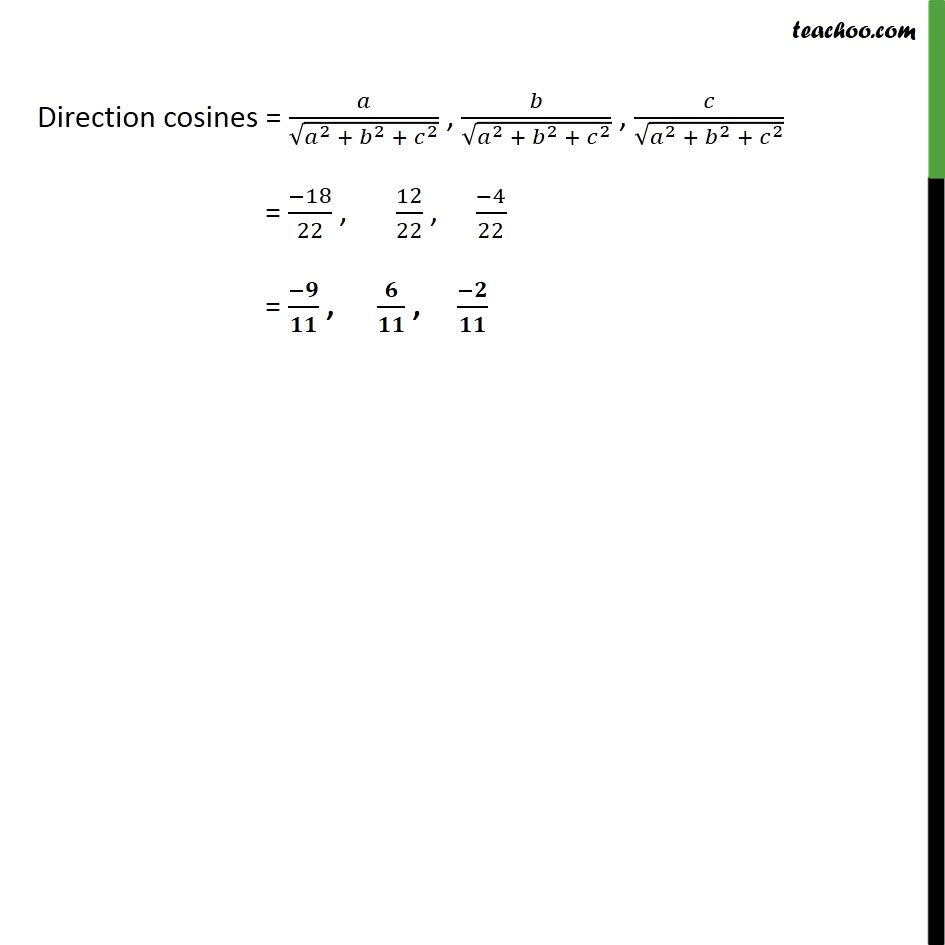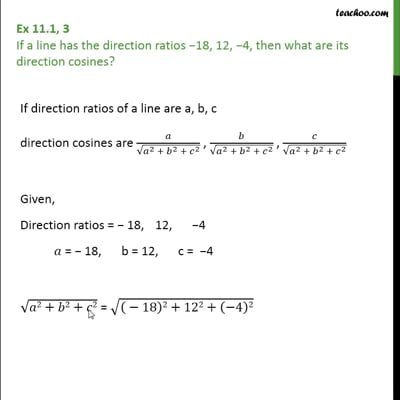Ex 11.1

Chapter 11 Class 12 Three Dimensional Geometry
Serial order wiseThis video is only available for Teachoo black users

Solve all your doubts with Teachoo Black (new monthly pack available now!)

### Transcript

Ex 11.1, 3 If a line has the direction ratios 18, 12, 4, then what are its direction cosines? If direction ratios of a line are a, b, c direction cosines are 2 + 2 + 2 , 2 + 2 + 2 , 2 + 2 + 2 Given, Direction ratios = 18, 12, 4 = 18, b = 12, c = 4 2+ 2+ 2 = 18 2+122+ 4 2 = 324+144+16 = 484 = 22 Direction cosines = 2 + 2 + 2 , 2 + 2 + 2 , 2 + 2 + 2 = 18 22 , 12 22 , 4 22 = , ,